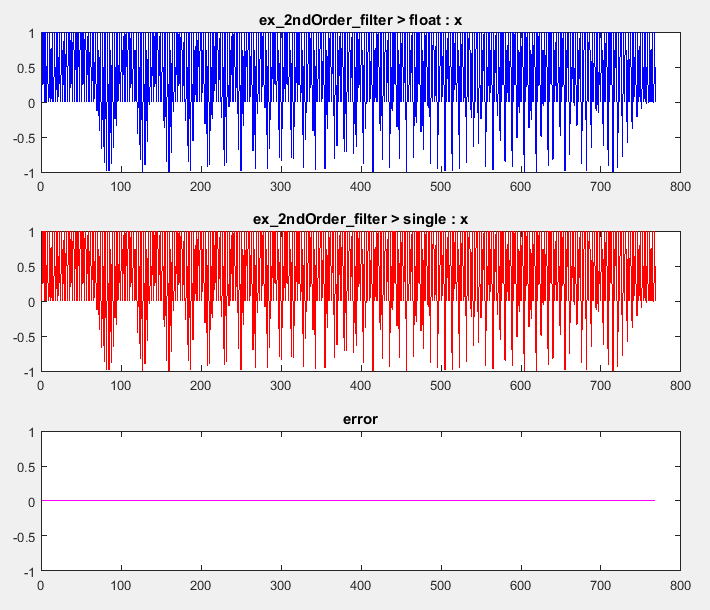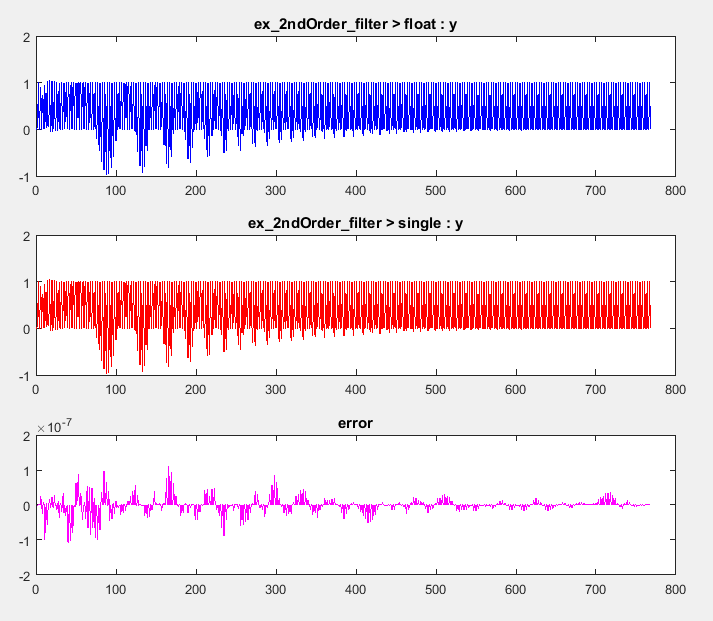## Generate Single-Precision MATLAB Code

This example shows how to generate single-precision MATLAB® code from double-precision MATLAB code. This example shows the single-precision conversion workflow that you use when you want to see single-precision MATLAB code or use verification options. Optionally, you can also generate single-precision C/C++ code.

### Prerequisites

To complete this example, install the following products:

• MATLAB

• MATLAB Coder™

• Fixed-Point Designer™

• C compiler

You can use `mex -setup` to change the default compiler. See Change Default Compiler.

### Create a Folder and Copy Relevant Files

1. In a local, writable folder, create a function `ex_2ndOrder_filter.m`.

```function y = ex_2ndOrder_filter(x) %#codegen persistent z if isempty(z) z = zeros(2,1); end % [b,a] = butter(2, 0.25) b = [0.0976310729378175, 0.195262145875635, 0.0976310729378175]; a = [1, -0.942809041582063, 0.3333333333333333]; y = zeros(size(x)); for i = 1:length(x) y(i) = b(1)*x(i) + z(1); z(1) = b(2)*x(i) + z(2) - a(2) * y(i); z(2) = b(3)*x(i) - a(3) * y(i); end end ```
2. Create a test file, `ex_2ndOrder_filter_test.m`, to exercise the `ex_2ndOrder_filter` algorithm.

It is a best practice to create a separate test script for preprocessing and postprocessing such as:

• Setting up input values.

• Calling the function under test.

• Outputting the test results.

To cover the full intended operating range of the system, the test script runs the `ex_2ndOrder_filter` function with three input signals: chirp, step, and impulse. The script then plots the outputs.

```% ex_2ndOrder_filter_test % % Define representative inputs N = 256; % Number of points t = linspace(0,1,N); % Time vector from 0 to 1 second f1 = N/2; % Target frequency of chirp set to Nyquist x_chirp = sin(pi*f1*t.^2); % Linear chirp from 0 to Fs/2 Hz in 1 second x_step = ones(1,N); % Step x_impulse = zeros(1,N); % Impulse x_impulse(1) = 1; % Run the function under test x = [x_chirp;x_step;x_impulse]; y = zeros(size(x)); for i = 1:size(x,1) y(i,:) = ex_2ndOrder_filter(x(i,:)); end % Plot the results titles = {'Chirp','Step','Impulse'} clf for i = 1:size(x,1) subplot(size(x,1),1,i) plot(t,x(i,:),t,y(i,:)) title(titles{i}) legend('Input','Output') end xlabel('Time (s)') figure(gcf) disp('Test complete.')```
TypeNameDescription
Function code`ex_2ndOrder_filter.m`Entry-point MATLAB function
Test file`ex_2ndOrder_filter_test.m`

MATLAB script that tests `ex_2ndOrder_filter.m`

### Set Up the Single-Precision Configuration Object

Create a single-precision configuration object. Specify the test file name. Verify the single-precision code using the test file. Plot the error between the double-precision code and single-precision code. Use the default values for the other properties.

```scfg = coder.config('single'); scfg.TestBenchName = 'ex_2ndOrder_filter_test'; scfg.TestNumerics = true; scfg.LogIOForComparisonPlotting = true;```

### Generate Single-Precision MATLAB Code

To convert the double-precision MATLAB function, `ex_2ndOrder_filter`, to single-precision MATLAB code, use the `codegen` function with the `-double2single` option.

`codegen -double2single scfg ex_2ndOrder_filter`

`codegen` analyzes the double-precision code. The conversion process infers types by running the test file because you did not specify the input types for the `ex_2ndOrder_filter` function. The conversion process selects single-precision types for the double-precision variables. It selects `int32` for index variables. When the conversion is complete, `codegen` generates a type proposal report.

### View the Type Proposal Report

To see the types that the conversion process selected for the variables, open the type proposal report for the `ex_2ndOrder_filter` function. Click the link `ex_2ndOrder_filter_report.html`.

The report opens in a web browser. The conversion process converted:

• Double-precision variables to `single`.

• The index `i` to `int32`. The conversion process casts index and dimension variables to `int32`.### View Generated Single-Precision MATLAB Code

To view the report for the generation of the single-precision MATLAB code, in the Command Window:

1. Scroll to the `Generate Single-Precision Code` step. Click the View report link.

2. In the MATLAB Source pane, click `ex_2ndOrder_filter_single`.

The code generation report displays the single-precision MATLAB code for `ex_2ndOrder_filter`.

### View Potential Data Type Issues

When you generate single-precision code, `codegen` enables highlighting of potential data type issues in code generation reports. If `codegen` cannot remove a double-precision operation, the report highlights the MATLAB expression that results in the operation. Click the Code Insights tab. The absence of potential data type issues indicates that no double-precision operations remain.

### Compare the Double-Precision and Single-Precision Variables

You can see the comparison plots for the input `x` and output `y` because you selected to log inputs and outputs for comparison plots .### Optionally Generate Single-Precision C Code

If you also want to generate single-precision C code, create a code configuration object for C code generation. Use this configuration object with the `-config` option of the `codegen` function. For example:

1. Create a code configuration object for generation of a C static library.

```cfg = coder.config('lib'); ```

2. Generate the C code. Enable generation of the code generation report.

`codegen -double2single scfg -config cfg ex_2ndOrder_filter -report`
3. To view the code generation report for the C code generation, click the View Report link.

In the Generated Code pane, click `ex_2ndOrder_filter.c`.

• Double-precision variables have type `float` in the C code.

• The index `i` is an integer.

When you generate single-precision code, `codegen` enables highlighting of potential data type issues in the code generation report. If `codegen` cannot remove a double-precision operation, the report highlights the MATLAB expression that results in the operation.

Click the Code Insights tab. Then, expand Potential data type issues. The absence of double-precision operations indicates that no double-precision operations remain.